# Push-Pull Buck Converter in Discontinuous Conduction Mode

This example shows how to control the output voltage of a push-pull buck converter. The current flowing through the inductor reaches zero during the switch off cycle of the MOSFETs and therefore the DC-DC converter operates in Discontinuous Conduction Mode (DCM). This mode of conduction is mostly used for low-power applications. To convert and maintain the input DC voltage as a nominal output voltage, the PI Controller subsystem uses a simple integral control. During startup, the reference voltage is ramped up to the desired output voltage.

The converter operates in DCM only if

•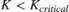,

where:

•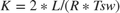.

•.

•is the filter inductance.

•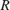is the load resistance.

•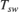is the switching period for each MOSFET. That is,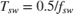, where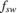is the switching frequency.

•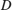is the duty cycle of PWM input to the gate of each MOSFET. That is,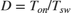, where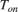is the ON time of the MOSFET.

### Open Model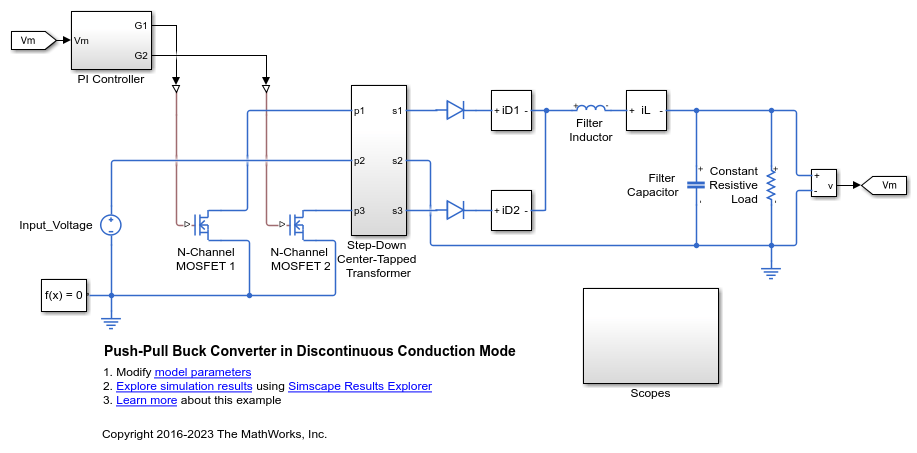### Specify the Design Parameters

The system is required to generate and maintain an output voltage of 80 V with a power requirement of 100 W. The input voltage is 400 V and the transformer turns ratio is 2. The load includes a constant resistive load. The 'PushPullBuckDCMData.m' script defines the design parameters as variables in the MATLAB® workspace.

### Operating Boundary Between Discontinuous Mode of Conduction and Continuous Mode of Conduction

Based on the constraint established by K and the Duty Cycle, the operating mode switches between Discontinuous Conduction Mode (DCM) and Continuous Conduction Mode (CCM). The Output Voltage Ratios of CCM and DCM are:

• Continuous Conduction Mode

•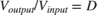• Discontinuous Conduction Mode

•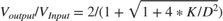### Calculate the Open-Loop Duty Cycle for DCM

The operating modes can be visualised for different values of K by generating a plot between the Output Voltage Ratio and the Duty Cycle. From this plot, find the corresponding Duty Cycle needed to achieve the Output Voltage Ratio as specified in the Design Parameters for the particular value of K chosen.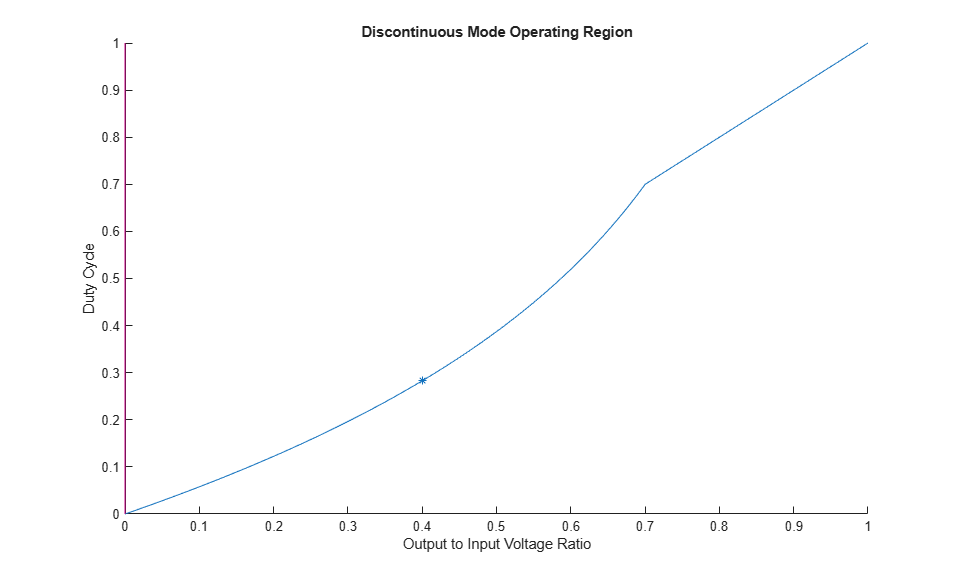### Determine the Filter Inductance

To estimate the inductance required for DCM, use this relationship between K, Resistance, and the Switching Time Period.

Verify the estimated inductance values result in DCM operation. If the value results in CCM operation, choose a different value of K and recalculate. Iterate until you find an inductance value that results in DCM operation.

### Choose a Filter Capacitance

The relation between the capacitance and the Output Voltage Ripple is

### Plot Capacitance Versus Voltage Ripple

Generate a plot to see the capacitance that is required for limiting the output voltage ripple varies depending on the design parameters. For this example, the marker at 1% Output Voltage Ripple corresponds to a capacitance of 8.157e-06 F.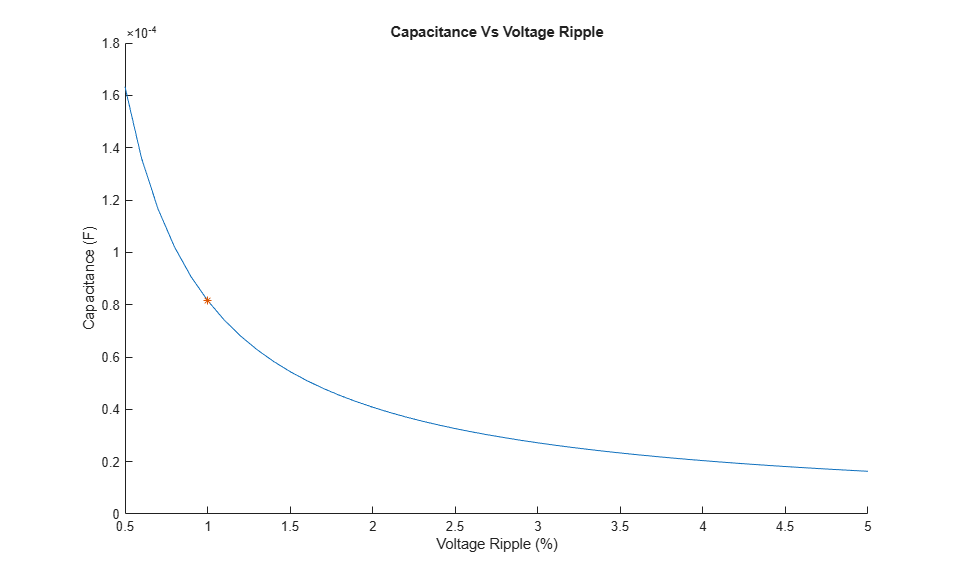### View Simulation Results

To view summary results during or after the simulation, open the Circuit Scope block from the Scopes subsystem or by entering, at the MATLAB command prompt: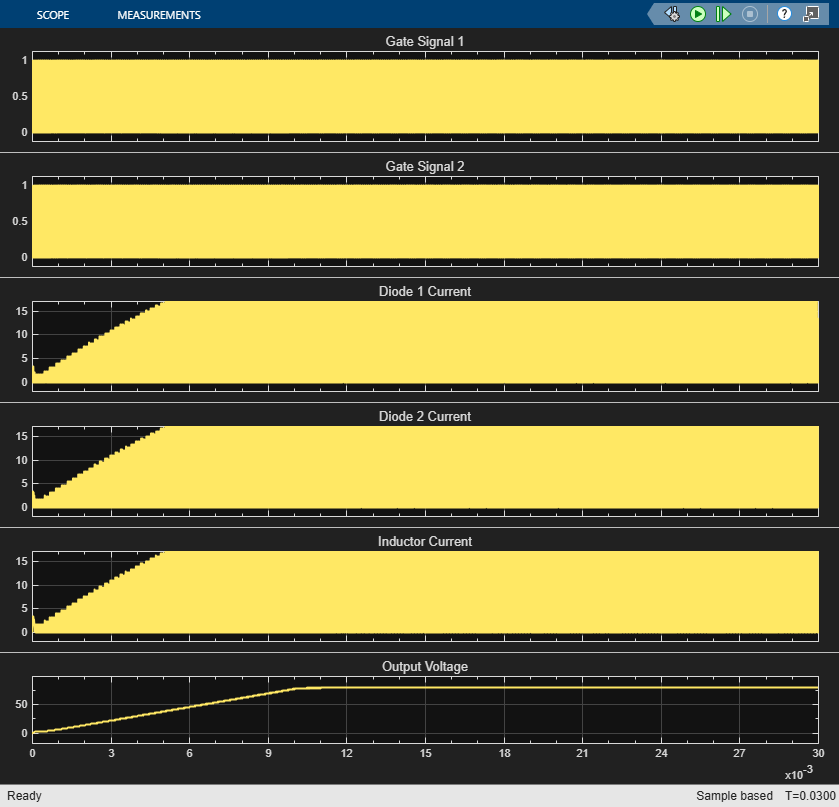To view the control and error data during or after the simulation, open the PI Controller Scope block from the Scopes subsystem or, enter: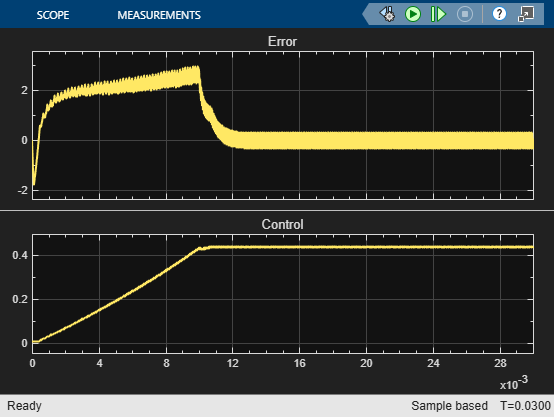After the simulation, to view logged Simscape™ data using the Simscape Results Explorer, enter: